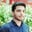Related Tags

pandas
python

# How to use the pandas.melt() function to unpivot a DataFrameSalman Yousaf

## Overview

The pandas library is used to manipulate data and is specifically for deep data analysis in Python. It comes with a bundle of pre-defined methods to perform statistical as well as mathematical operations on any type of data.

### What is pandas.melt()?

The pandas.melt() function is used to unpivot a DataFrame from wide to long format. This function is used to melt a DataFrame into one or more column identifier variables, (id variables), where other columns can be measured as value variable,s (val variables).

### Syntax

pandas.melt(frame,
id_vars=None,
value_vars=None,
var_name=None,
value_name='value',
col_level=None,
ignore_index=True)

### Parameters

It takes the below-listed argument values.

• frame: This is a DataFrame.
• id_vars: These are columns used as identifier variables. It can be either a list, tuple, or ndarray. The default value is None.
• value_vars: This is the column or columns to unpivot. If None is passed, it will include all columns that are not specified as id_vars.
• var_name: If None is specified, the default variable name will be variable or frame.columns.name. Otherwise, argument variable names are used for columns.
• value_name: This is the name to be used for the value column. By default, it's value.
• col_level: This helps to melt multi-index columns. By default, it is None.

### Return value

This method returns an unpivoted DataFrame.

### Explanation

In the following code snippet, we have a DataFrame named df where id_vars is ['A'] column and value_vars is ['B', 'C'].

import pandas as pd
# creating a DataFrame
df = pd.DataFrame({'A': {0: 'x', 1: 'y', 2: 'z'},
'B': {0: 10, 1: 20, 2: 30},
'C': {0: 40, 1: 50, 2: 60}})
print("DataFrame")
print(df)
# Invoking melt() to reshape it
melted_df= pd.melt(df, id_vars=['A'], value_vars=['B', 'C'])
#print melted df
print("Melted DataFrame")
print(melted_df)
• Lines 3–7: There are multiple ways to create a DataFrame, but in this line, we pass a Python dictionary to pd.DataFrame() to convert it into a DataFrame df, such as 3x3.
• Line 9: We reshape df from a wide to a long format in this line. The pd.melt() function will transform this data frame into an ID-value pair. Column 'A' will act as the ID variable, the id_vars column, while columns 'B', 'C' will act as value variables, the value_vars column.
• Lines 10–13: We will print a melted DataFrame of six entries (6x3) because Columns 'B' and 'C' are acting as values.

RELATED TAGS

pandas
python

CONTRIBUTORSalman Yousaf
RELATED COURSES

View all Courses

Keep Exploring

Learn in-demand tech skills in half the time